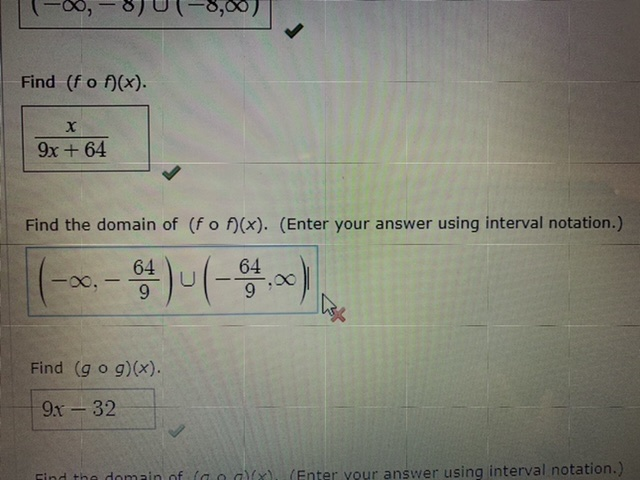# Find (fo f)(x). х 9x + 64 Find the domain of (fo f)(x). (Enter your answer using interval notation.) 64 00 Find (g o g)(x). 9x-32 Find the domain of (a o g(x). (Enter vour answer using interval notation.)

Question

f(x)=x/x+8 , g(x)=3x-8

I don't understand why my answer is incorrect, what am I doing wrong?help_outlineImage TranscriptioncloseFind (fo f)(x). х 9x + 64 Find the domain of (fo f)(x). (Enter your answer using interval notation.) 64 00 Find (g o g)(x). 9x-32 Find the domain of (a o g(x). (Enter vour answer using interval notation.) fullscreen

### Want to see this answer and more?

Experts are waiting 24/7 to provide step-by-step solutions in as fast as 30 minutes!*

*Response times may vary by subject and question complexity. Median response time is 34 minutes for paid subscribers and may be longer for promotional offers.
Tagged in
Math
Algebra

### Other